# Linear Predictive Coding (LPC)

## Introducing Remarks

• In Speech Coding the properties of speech have to be considered (unlike channel coding)
• For telephone applications the speech signal has to be re-synthesized (unlike speech recognition)
• Frames with a length of 10-30ms are considered
• The signal is considered stationary within a frame
• The LPC coefficients are calculated for each such frame
• The synthesized signal can be re-used
• Analysis-by-Synthesis sytem
•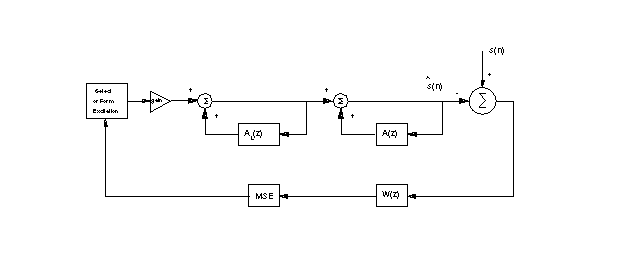## The Analysis-by-Synthesis System

• A short-term LP synthesis filter representing spectral information of the speech signal
• A long-term LP synthesis filter representing the pitch structure (optional)
• A perceptual weighting filter, shaping the error in such a way that the quantization noise is masked by high-energy formants
• Mean Squared Error (MSE) which minimizes the error signal
• An excitation source, which is selected according to the error signal
• The excitation is either white noise (unvoiced) or a uniform sample train (voiced)
• Assume that q=0 ( autoregressive or AR model), i.e. any zeros are ignored, since they only add linear phase
• Speech s(n) is filtered by an invers or predictor filter of an all-pole H(z)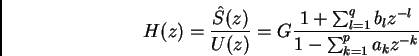(1)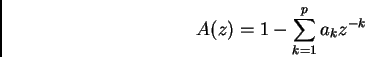(2)

• and the output e(n) is called error or risidual signal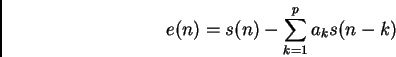(3)

## Least-Squares Autocorrelation Method

• The classical least-squares method minimizes the mean energy in the error signal over a frame of speech data
• The speech signal is multiplied by a Hamming window (or similar window)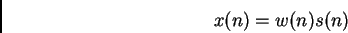(4)

• The LPC coefficients describe a smoothed average of the signal
• Let E be the error energy(5)

where e(n) is the risidual corresponding to the windowed signal x(n)

• The coefficients are found by partial differentiations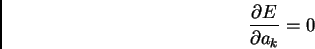(6)

This yields p linear equations in p unknown filter coefficients

• Finally we receive the minimum risidual energy or prediction energy for a p-pole model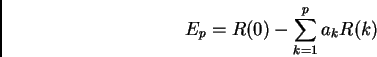(7)

### Problems

There are only two options for excitation which lowers speech quality